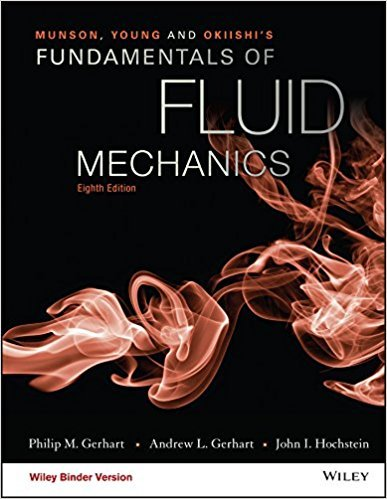×
×

# Figure P4.64 shows a fixed control volume. It has a volumeV0 = 1.0 ft3, a flow area A =ISBN: 9781119080701 456

## Solution for problem 4.64 Chapter 4.4

Fundamentals of Fluid Mechanics | 8th Edition

• Textbook Solutions
• 2901 Step-by-step solutions solved by professors and subject experts
• Get 24/7 help from StudySoup virtual teaching assistantsFundamentals of Fluid Mechanics | 8th Edition

4 5 1 398 Reviews
14
1
Problem 4.64

Figure P4.64 shows a fixed control volume. It has a volumeV0 = 1.0 ft3, a flow area A = 1.0 ft2, and a length 0 = 1.0 ft.Position x represents the center of the control volume where thefluid velocity V0 = 1.0 ft/s and the density 0 = 1.800 slug/ft3.Also, at position x the fluid density does not change locally withtime but decreases in the axial direction at the linear rate of0.25 slug/ft4. Use the system or Lagrangian approach to evaluatedp/dt. Compare this result with that of the material derivative andflux terms.

Step-by-Step Solution:
Step 1 of 3

Chapter 1 1.1 Chemistry and Chemicals  Chemistry is the study the composition, structure, properties, and reactions of matter o Matter is another word for all the substances that make up our world.  The field of chemistry is divided into several branches. o General chemistry is the study of the composition, properties, and reactions...

Step 2 of 3

Step 3 of 3

##### ISBN: 9781119080701

Unlock Textbook Solution